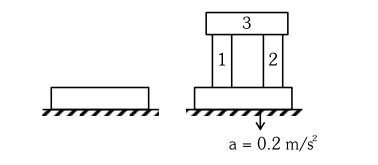# A steel block of 10kg

Question:

A steel block of $10 \mathrm{~kg}$ rests on a horizontal floor as shown. When three iron cylinders are placed on it as shown, the block and cylinders go down with an acceleration $0.2 \mathrm{~m} / \mathrm{s}^{2}$. The normal reaction $R^{\prime}$ by the floor if mass of the iron cylinders are equal and of $20 \mathrm{~kg}$ each, is

N. [Take $g=10 \mathrm{~m} / \mathrm{s}^{2}$ and $\left.\mu_{\mathrm{s}}=0.2\right]$1. 716

2. 686

3. 714

4. 684

Correct Option: , 2

Solution:

Writing force equation in vertical direction

$\mathrm{Mg}-\mathrm{N}=\mathrm{Ma}$

$\Rightarrow 70 \mathrm{~g}-\mathrm{N}=70 \times 0.2$

$\Rightarrow \mathrm{N}=70[\mathrm{~g}-0.2]=70 \times 9.8$

$\therefore \mathrm{N}=686$ Newton

Note : Since there is no compressive normal from the sides, hence friction will not act.

Hence option 2 .